GMAT Math : DSQ: Calculating arithmetic mean

Example Questions

1 3 Next →

Example Question #41 : Descriptive Statistics

Given five distinct positive integers -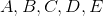- which of them is the median?

Statement 1: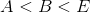Statement 2: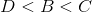STATEMENT 2 ALONE provides sufficient information to answer the question, but STATEMENT 1 ALONE does NOT provide sufficient information to answer the question.

EITHER STATEMENT ALONE provides sufficient information to answer the question.

BOTH STATEMENTS TOGETHER do NOT provide sufficient information to answer the question.

BOTH STATEMENTS TOGETHER provide sufficient information to answer the question, but NEITHER STATEMENT ALONE provides sufficient information to answer the question.

STATEMENT 1 ALONE provides sufficient information to answer the question, but STATEMENT 2 ALONE does NOT provide sufficient information to answer the question.

BOTH STATEMENTS TOGETHER provide sufficient information to answer the question, but NEITHER STATEMENT ALONE provides sufficient information to answer the question.

Explanation:

The median of five numbers (an odd number) is the number in the middle when they are arranged in ascending order.

These two orderings are both consistent with the ordering given in Statement 1: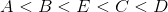- median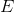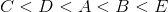- median.

Therefore, Statement 1 alone provides insufficient information to answer the question. For a similar reason, so does Statement 2.

Assume both statements to be true. Thenis greater than bothandand less than bothand. That makesthe middle element, and, thus, the median.

Example Question #42 : Descriptive Statistics

Give the arithmetic mean ofand.

Statement 1: A rectangle with lengthand widthhas area 500.

Statement 2: A triangle with base of lengthand heighthas area 250.

BOTH STATEMENTS TOGETHER provide sufficient information to answer the question, but NEITHER STATEMENT ALONE provides sufficient information to answer the question.

EITHER STATEMENT ALONE provides sufficient information to answer the question.

STATEMENT 1 ALONE provides sufficient information to answer the question, but STATEMENT 2 ALONE does NOT provide sufficient information to answer the question.

BOTH STATEMENTS TOGETHER do NOT provide sufficient information to answer the question.

STATEMENT 2 ALONE provides sufficient information to answer the question, but STATEMENT 1 ALONE does NOT provide sufficient information to answer the question.

BOTH STATEMENTS TOGETHER do NOT provide sufficient information to answer the question.

Explanation:

The area of a rectangle is the product of its length and width; the area of a triangle is half the product of its height and the length of its base. Therefore, from Statement 1, we get that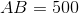.

From Statement 2, we get that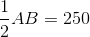, or, equivalently,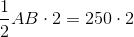, orIn other words, the two statements are equivalent, so one of two things happens - either statement alone is sufficient, or both together are insufficient. We show that the latter is the case:

Case 1: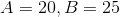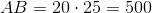The mean of the two is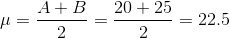.

Case 2: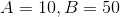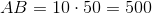The mean of the two is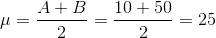.

Therefore, knowing the area of the rectangle with these dimensions is not helpful to determining their arithmetic mean. This makes Statement 1, and, equivalently, both statements together, unhelpful.

Example Question #43 : Descriptive Statistics

Joseph's final grade is calculated from the mean of his test scores. His teacher also allows them to drop the lowest score before calculating the final grade. If Joseph received a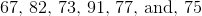on his tests, what was his final grade rounded to the nearest whole number?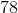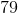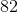Explanation:

The average or mean is found by taking all of the scores and dividing by the total number of scores. Remember, we must first find the lowest score and not include that in the calculation. Therefore, we get: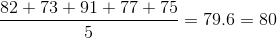when rounded.

Example Question #44 : Descriptive Statistics

You are given the data set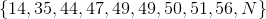, whereis an integer not necessarily greater than 56. What is the value of?

Statement 1: The mean of the data set is 44.3

Statement 2: The median of the data set is 48.5

Statement 1 ALONE is sufficient to answer the question, but Statement 2 ALONE is NOT sufficient to answer the question.

Statement 2 ALONE is sufficient to answer the question, but Statement 1 ALONE is NOT sufficient to answer the question.

BOTH statements TOGETHER are insufficient to answer the question.

BOTH statements TOGETHER are sufficient to answer the question, but NEITHER statement ALONE is sufficient to answer the question.

EITHER statement ALONE is sufficient to answer the question.

EITHER statement ALONE is sufficient to answer the question.

Explanation:

Knowing the mean of the set is enough to calculate: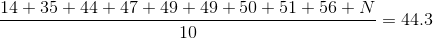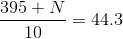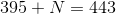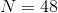Knowing the median is enough to deduce. Since there are ten elements - an even number - the median is the arithmetic mean of the fifth and sixth highest elements. If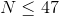, those two elements are 47 and 49, making the median 48. If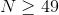, those two elements are both 49, making the median 49. This forcesto be 48, making the median 48.5.

Therefore, either statement alone is sufficient to answer the question.

1 3 Next →

Tired of practice problems?

Try live online GMAT prep today.